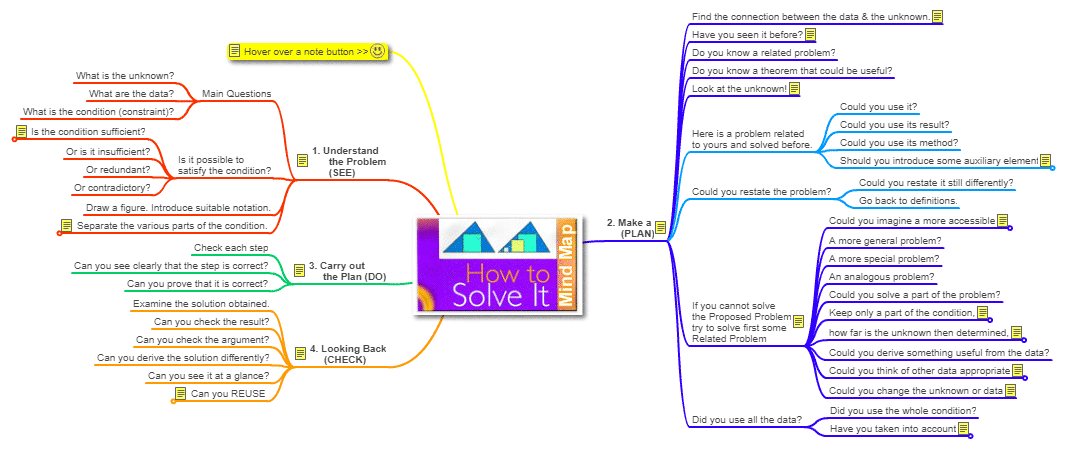# Mind Map: How to Solve It by George Polya, A new aspect of Mathematical MethodGeorge Pólya's 1945 book How to Solve It, A new aspect of Mathematical Method, is a book describing methods of problem solving. It suggests the following steps when solving a mathematical problem: First, you have to understand the problem. After understanding, then make a plan. Carry out the plan. Look back on your work. How could it be better? Polya's work has had a large influence on mathematics textbooks. "Most formulations of a problem solving framework in U. S. textbooks attribute some relationship to Polya's problem solving stages (1945)."     Last updated: December 19, 2010.
 Home | Mind Maps | Geometry | Academic Disciplines | Problem Solving Techniques | Email